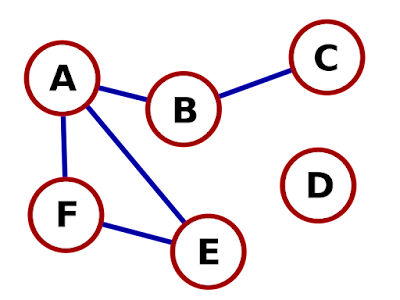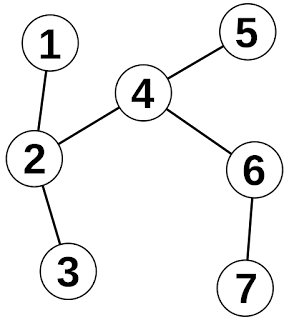# What are Graphs and its Types in Data Structure

A graph represents a collection of objects, with some pairs of things connected by links. The points that connect the connected objects are called vertices, and the ties that bind the vertices are called edges.

A graph is defined as a pair of sets (V, E), where V represents the set of vertices and E represents the set of edges that connect the pairs of vertices. For example, take a look at the graph below.In the above graph A, B, C, D, and E are known as Vertices, and ( AB, BC, AF, FE, and AE) are known as Edges.

Graphs Data Structure

They can represent mathematical graphs in a data structure and algorithms. We can define a graph using an array of vertices and a two-dimensional array of edges. Before we proceed further, let's familiarize ourselves with some essential terms −

Vertex: Each node of the Graph is represented as a vertex. In the following example, the labeled circle represents vertices. Thus, A to G are vertices. We can describe them using an array, as shown in the following image. Here A can be identified by index 0. B can be identified using index one and so on.

Edge: The edge represents a path between two vertices or a line between two vertices. In the following example, lines A to B and B to C represent edges. As shown in the following image, we can use a two-dimensional array to define an array. Here AB can be described as one at row 0, column 1, BC as one at row 1, column 2, and so on, keeping other combinations as 0.

Adjacency: Two nodes or vertices are adjacent if connected through an edge. In the following example, B is adjacent to A, C is adjacent to B, etc.

Path: The path represents a sequence of edges between the two vertices. In the following example, ABCD represents a path from A to D.Basic Operations

Following are the basic primary operations of a Graph −

Add Edge: Adds an edge between the two vertices of the Graph.
Display Vertex: Displays a vertex of the Graph.

Types of Graphs in Data Structures

There are various types of graphs in data structure:

1. Finite Graph
2. Infinite Graph
3. Trivial Graph
4. Simple Graph
5. Multi Graph
6. Null Graph
7. Complete Graph
8. Pseudo Graph
9. Regular Graph
10. Weighted Graph
11. Directed Graph
12. Undirected Graph
13. Connected Graph
14. Disconnected Graph
15. Cyclic Graph
16. Acyclic Graph
17. Directed Acyclic Graph
18. Subgraph Graph

To know more about graphs and data structures and algorithms, please read Graph Theory Tutorial. We shall learn about traversing a graph in the coming chapters.